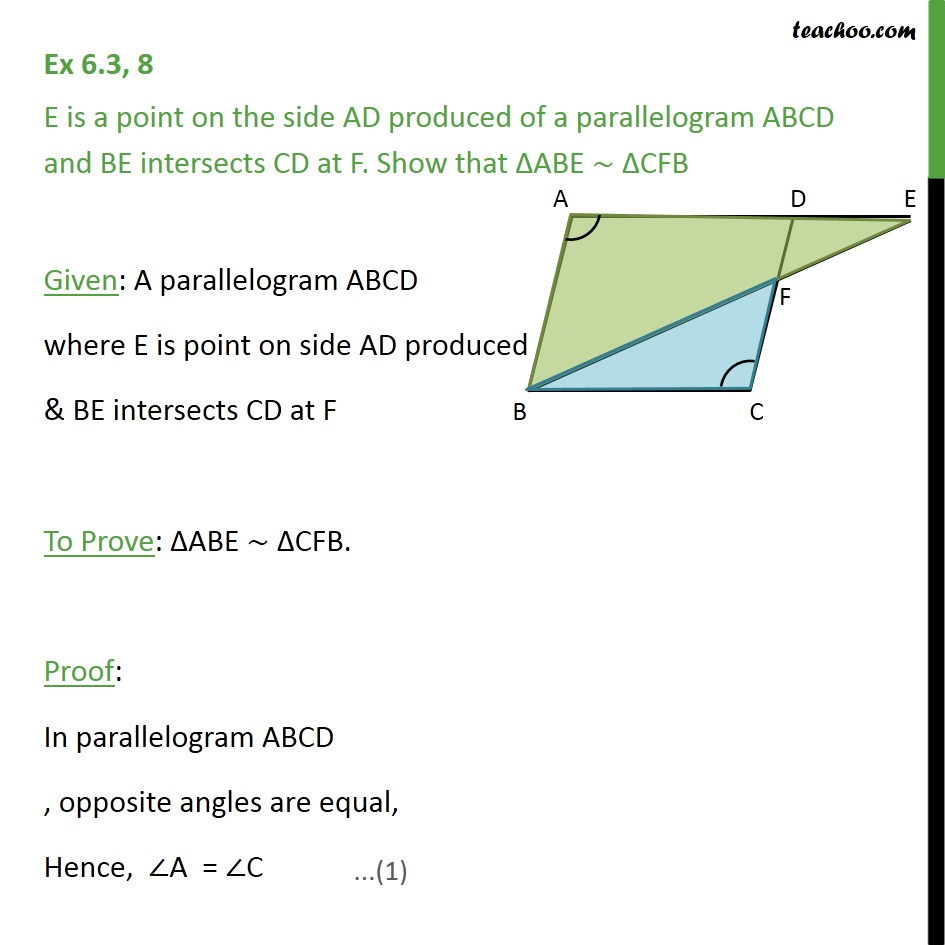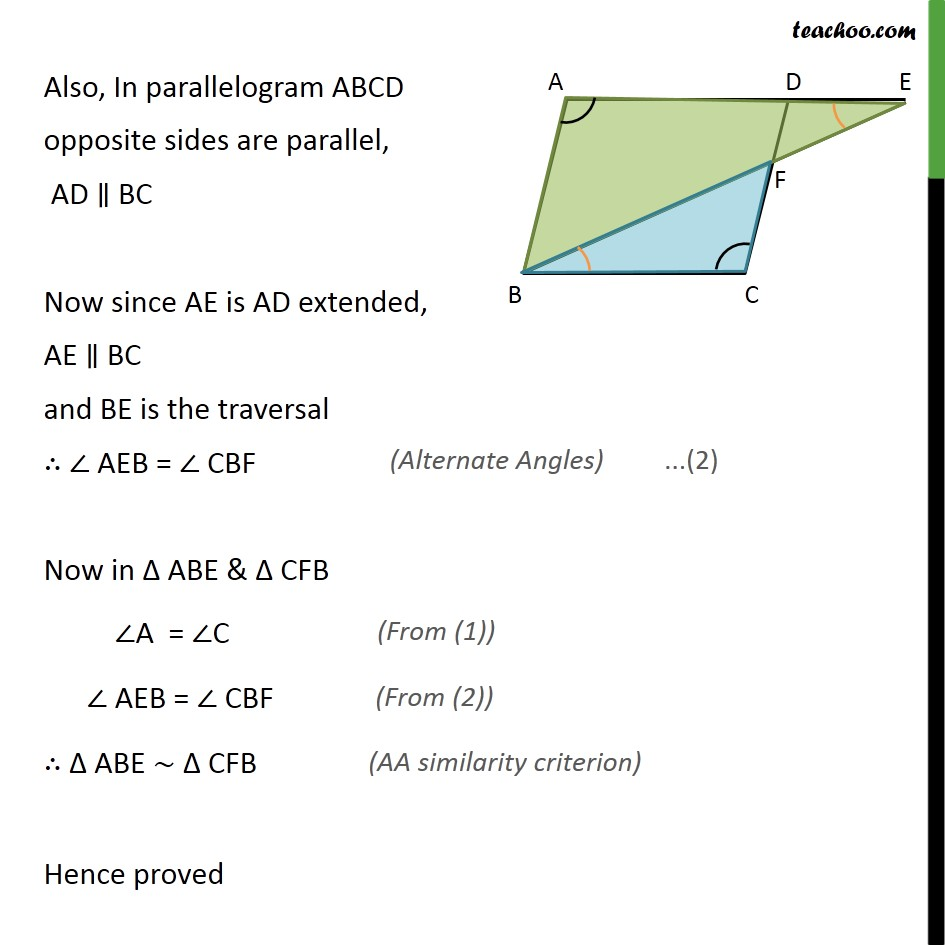Ex 6.3

Chapter 6 Class 10 Triangles
Serial order wiseGet live Maths 1-on-1 Classs - Class 6 to 12

### Transcript

Ex 6.3, 8 E is a point on the side AD produced of a parallelogram ABCD and BE intersects CD at F. Show that ΔABE ∼ ΔCFB Given: A parallelogram ABCD where E is point on side AD produced & BE intersects CD at F To Prove: ΔABE ∼ ΔCFB. Proof: In parallelogram ABCD , opposite angles are equal, Hence, ∠A = ∠C Also, In parallelogram ABCD opposite sides are parallel, AD ∥ BC Now since AE is AD extended, AE ∥ BC and BE is the traversal ∴ ∠ AEB = ∠ CBF Now in Δ ABE & Δ CFB ∠A = ∠C ∠ AEB = ∠ CBF ∴ Δ ABE ∼ Δ CFB Hence proved